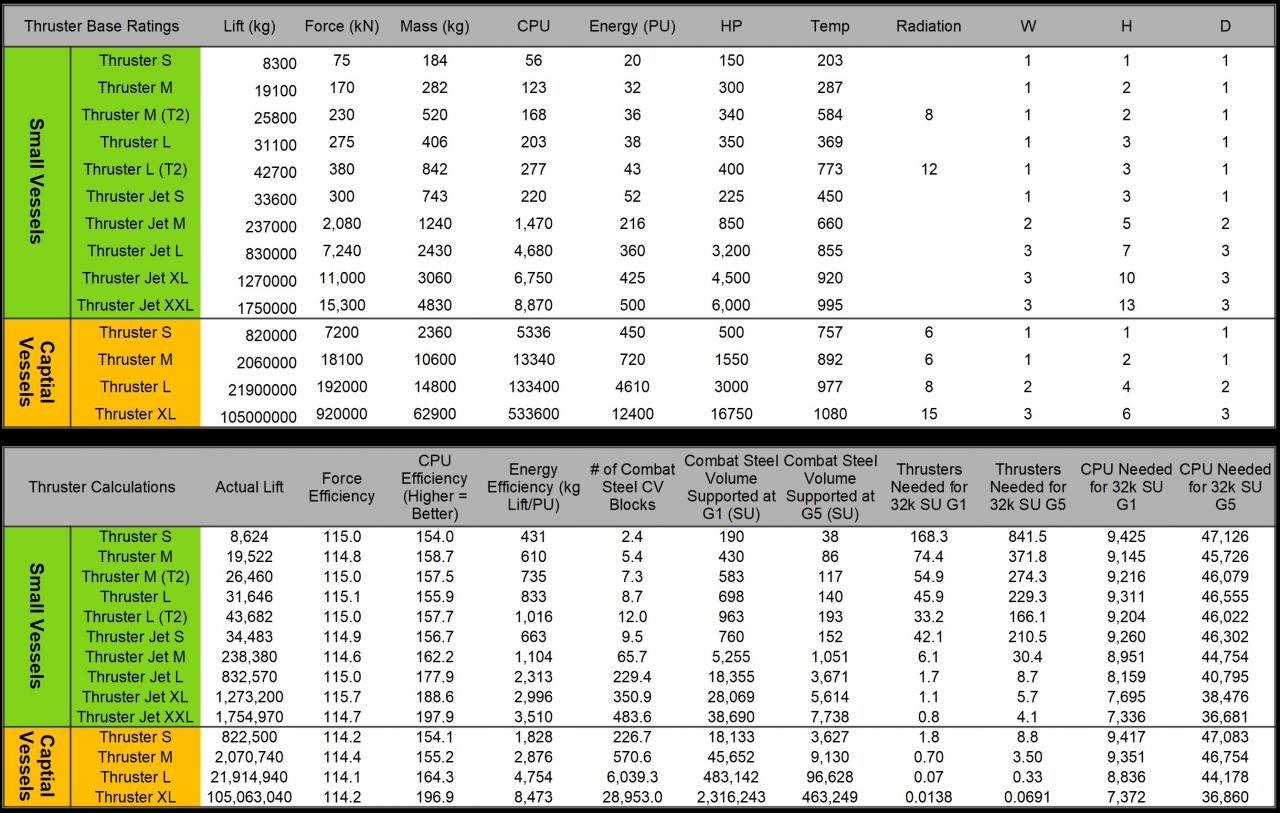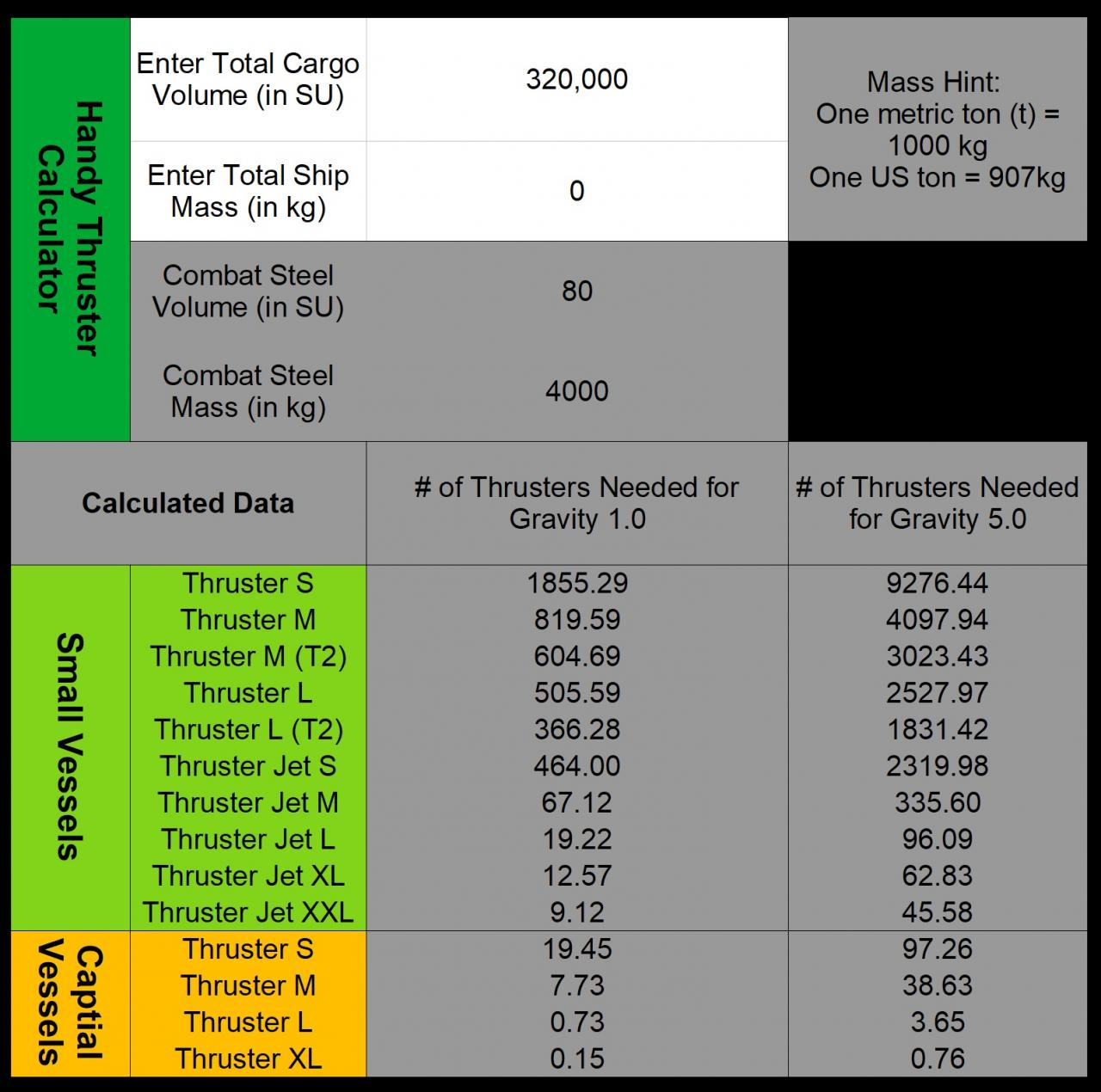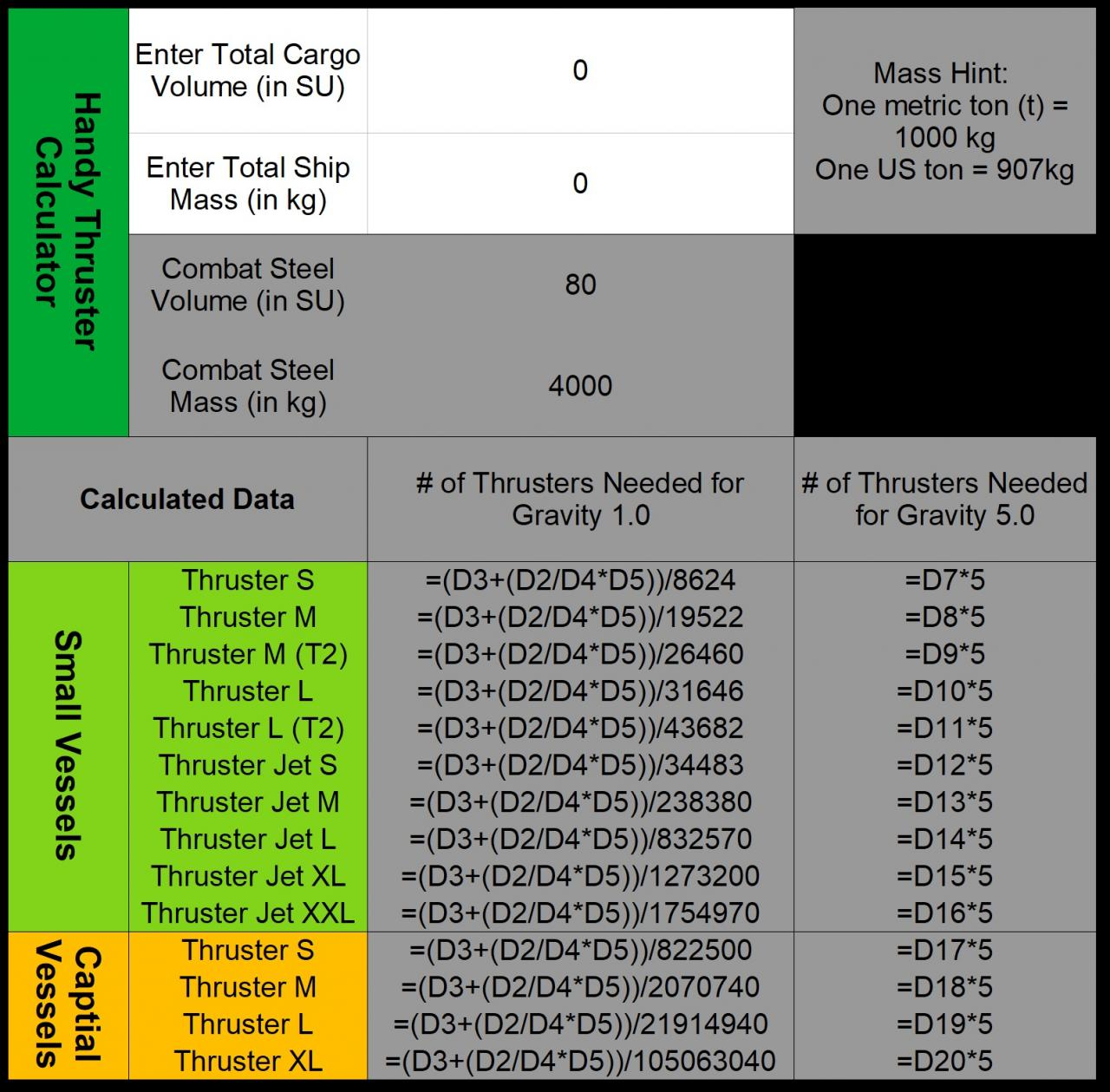# Empyrion Galactic Survival: Engineer’s Guide on Thruster Engines

Is your ship sinking in atmosphere? May be time to contact the engineer responsible for thrusters… or you could just read this guide!

### Engineer’s Journal concerning Thruster Engines(click image for better resolution)

Explanation:

Approximate calculations were made for SV and CV thruster engines to help guide you on ship building. All thrusters were tested using only a core and each thruster to get a close to accurate readings as possible.

Then those results were used to calculate the bottom half of the table. Which has useful information, such as how many engines it would take to support a 32k cargo controller full of combat steel in 1.0 Gravity or 5.0 Gravity environments.

I have started to use this myself when trying to figure out if I will have enough thrust for my ship design. Enjoy!

***Caution***
Disclaimer: These are all based of rough calculations and only are for thrust in a single direction. Don’t forget the other 5 directions…although vertical lift and forward lift are usually most important.

### Handy Thruster Calculation Tool

I have created a handy spreadsheet to act as a calculator.. just key in your total storage and ship’s mass and it will give you the total county of thrusters needed if you wanted to only use small thrusters, or large. A combination of thrusters are not accounted for in this calculator.

(Example in left image, formulas shown in right image with D2 square being the Total Cargo entered)Want the above calculator to use yourself? Here is the csv text to make this spreadsheet yourself:

Code Beginning

Handy Thruster Calculator,Enter Total Cargo Volume (in SU),0,”Mass Hint: One metric ton (t) = 1000 kg One US ton = 907kg”
,Enter Total Ship Mass (in kg),0,
,Combat Steel Volume (in SU),80,
,Combat Steel Mass (in kg),4000,
Calculated Data,,# of Thrusters Needed for Gravity 1.0,# of Thrusters Needed for Gravity 5.0
Small Vessels,Thruster S,=(D3+(D2/D4*D5))/8624,=D7*5
,Thruster M,=(D3+(D2/D4*D5))/19522,=D8*5
,Thruster M (T2),=(D3+(D2/D4*D5))/26460,=D9*5
,Thruster L,=(D3+(D2/D4*D5))/31646,=D10*5
,Thruster L (T2),=(D3+(D2/D4*D5))/43682,=D11*5
,Thruster Jet S,=(D3+(D2/D4*D5))/34483,=D12*5
,Thruster Jet M,=(D3+(D2/D4*D5))/238380,=D13*5
,Thruster Jet L,=(D3+(D2/D4*D5))/832570,=D14*5
,Thruster Jet XL,=(D3+(D2/D4*D5))/1273200,=D15*5
,Thruster Jet XXL,=(D3+(D2/D4*D5))/1754970,=D16*5
Captial Vessels,Thruster S,=(D3+(D2/D4*D5))/822500,=D17*5
,Thruster M,=(D3+(D2/D4*D5))/2070740,=D18*5
,Thruster L,=(D3+(D2/D4*D5))/21914940,=D19*5
,Thruster XL,=(D3+(D2/D4*D5))/105063040,=D20*5

Code End

Instructions:
1) Copy and paste the above text (between Code Beginning & End) into a notpad file then save the file a name of your choice
2) Convert the file extension to a .csv file (may have to have extensions visible in your explorer).
3) Now you should be able to open this with most spreadsheet programs.

By Fuji